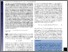How multiplicity determines entropy and the derivation of the maximum entropy principle for complex systems

Hanel, R., Thurner, S., & Gell-Mann, M. (2014). How multiplicity determines entropy and the derivation of the maximum entropy principle for complex systems. Proceedings of the National Academy of Sciences 111 (19) 6905-6910. 10.1073/pnas.1406071111.Preview
Text
How multiplicity determines entropy and the derivation of the maximum entropy principle for complex systems.pdf - Published Version

Abstract

The maximum entropy principle (MEP) is a method for obtaining the most likely distribution functions of observables from statistical systems by maximizing entropy under constraints. The MEP has found hundreds of applications in ergodic and Markovian systems in statistical mechanics, information theory, and statistics. For several decades there has been an ongoing controversy over whether the notion of the maximum entropy principle can be extended in a meaningful way to nonextensive, nonergodic, and complex statistical systems and processes. In this paper we start by reviewing how Boltzmann-Gibbs-Shannon entropy is related to multiplicities of independent random processes. We then show how the relaxation of independence naturally leads to the most general entropies that are compatible with the first three Shannon-Khinchin axioms, the (c,d)-entropies. We demonstrate that the MEP is a perfectly consistent concept for nonergodic and complex statistical systems if their relative entropy can be factored into a generalized multiplicity and a constraint term. The problem of finding such a factorization reduces to finding an appropriate representation of relative entropy in a linear basis. In a particular example we show that path-dependent random processes with memory naturally require specific generalized entropies. The example is to our knowledge the first exact derivation of a generalized entropy from the microscopic properties of a path-dependent random process.

Item Type: Article Thermodynamics; Out-of-equilibrium process; Driven systems; Random walk Advanced Systems Analysis (ASA) PNAS; 111(19):6905-6910 (13 May 2014) (Published online 29 April 2014) IIASA Import 15 Jan 2016 08:50 27 Aug 2021 17:39 http://pure.iiasa.ac.at/10922View Item